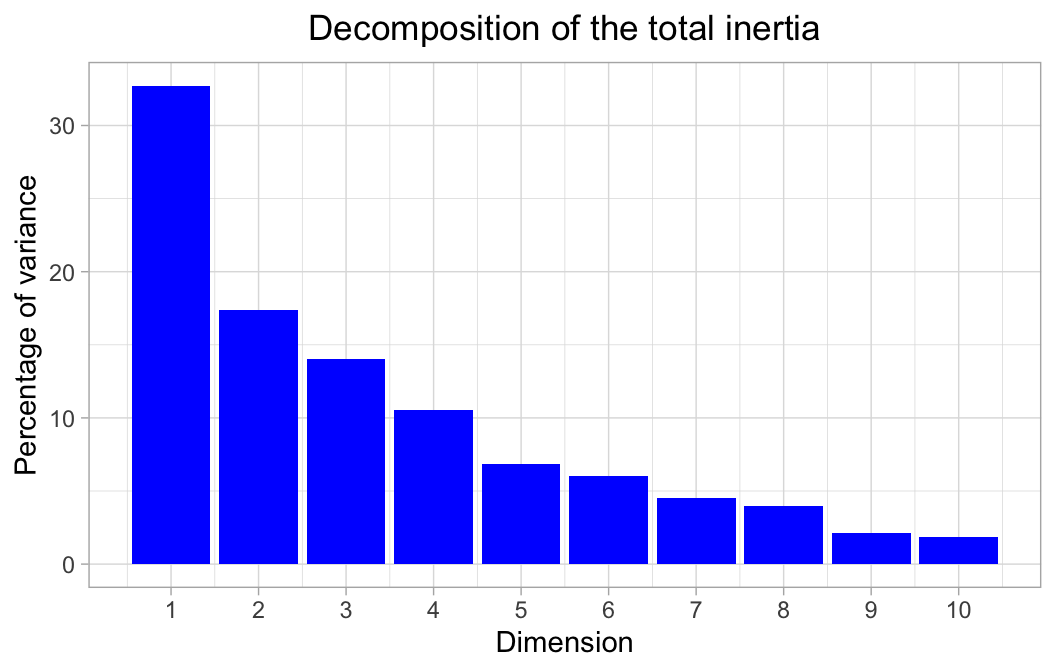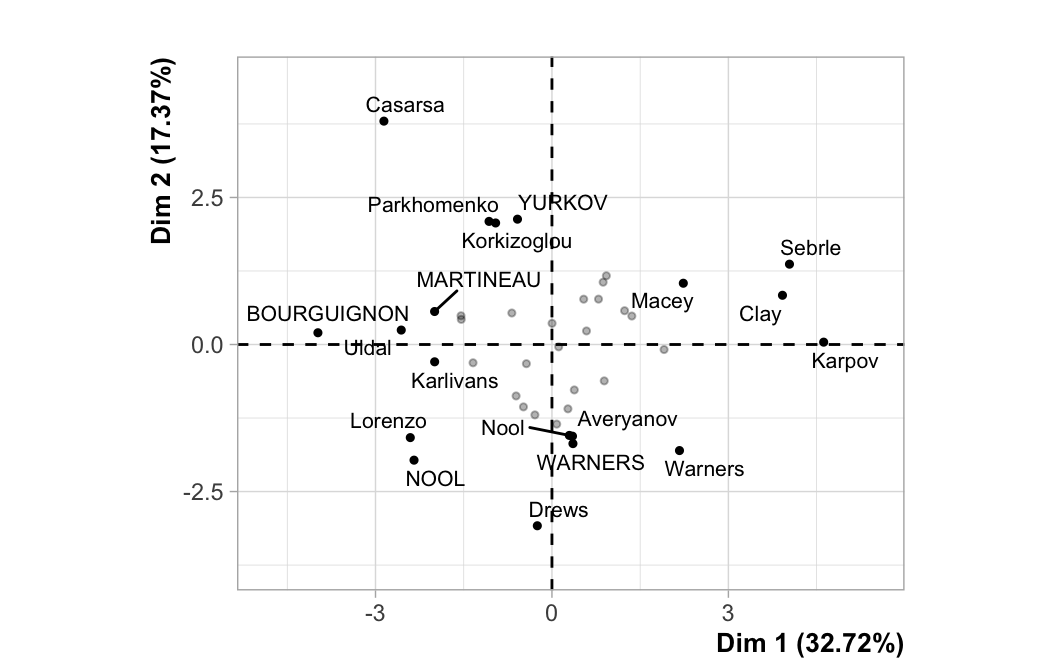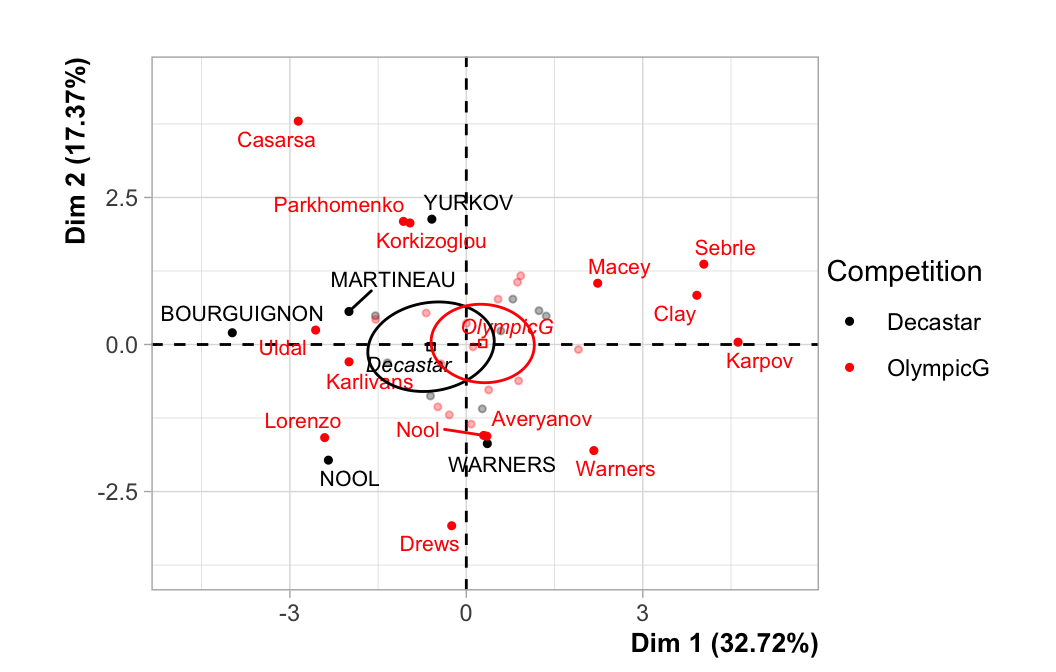This dataset contains 41 individuals and 13 variables, 2 quantitative variables are considered as illustrative, 1 qualitative variable is considered as illustrative.

### 1. Study of the outliers

The analysis of the graphs does not detect any outlier.

### 2. Inertia distribution

The inertia of the first dimensions shows if there are strong relationships between variables and suggests the number of dimensions that should be studied.

The first two dimensions of analyse express 50.09% of the total dataset inertia ; that means that 50.09% of the individuals (or variables) cloud total variability is explained by the plane. This percentage is relatively high and thus the first plane well represents the data variability. This value is greater than the reference value that equals 37.93%, the variability explained by this plane is thus significant (the reference value is the 0.95-quantile of the inertia percentages distribution obtained by simulating 1686 data tables of equivalent size on the basis of a normal distribution).

From these observations, it should be better to also interpret the dimensions greater or equal to the third one.Figure 2 - Decomposition of the total inertia

An estimation of the right number of axis to interpret suggests to restrict the analysis to the description of the first 3 axis. These axis present an amount of inertia greater than those obtained by the 0.95-quantile of random distributions (64.14% against 51.55%). This observation suggests that only these axis are carrying a real information. As a consequence, the description will stand to these axis.

### 3. Description of the plane 1:2Figure 3.1 - Individuals factor map (PCA) The labeled individuals are those with the higher contribution to the plane construction.

The Wilks test p-value indicates which variable factors are the best separated on the plane (i.e. which one explain the best the distance between individuals).

``````## Competition
##    0.366311``````

There only is one possible qualitative variable to illustrate the distance between individuals : Competition.Figure 3.2 - Individuals factor map (PCA) The labeled individuals are those with the higher contribution to the plane construction. The individuals are coloured after their category for the variable Competition.